StatLect

# Markov Chain Monte Carlo (MCMC) methods

Markov Chain Monte Carlo (MCMC) methods are very powerful Monte Carlo methods that are often used in Bayesian inference.

While "classical" Monte Carlo methods rely on computer generated samples made up of independent observations, MCMC methods are based on techniques that allow to generate sequences of dependent observations (these sequences are Markov chains, hence the name of the methods).

Throughout this lecture we are going to assume that you are familiar with:## How MCMC works

The purpose of any Monte Carlo method is to approximate some feature (e.g., the mean) of a given probability distribution. This is accomplished by using a computer generated sample of draws from the given distribution to compute a plug-in estimate of the feature to be approximated. In particular, suppose we are given a random vectorwith joint distribution function, and we want to approximate a featureof the distribution.

In a Markov Chain Monte Carlo method, we generate through a computer algorithm a sample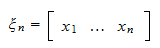of realizations ofrandom variables, ...,.

The algorithm is devised in such a way that the sequenceis a Markov Chain converging to the stationary distribution.

The sample is used, as in standard Monte Carlo methods, to produce a plug-in estimateof the feature, whereis the empirical distribution of the sample(i.e., a probability distribution that assigns probabilityto each one of the values).

Unlike in standard Monte Carlo methods, the variables, ...,are in general not independent. We need to take this fact into account when we assess the convergence ofto. For example, ifis the expected value of, andis the sample mean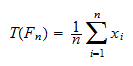we are usually able to show that an ergodic theorem (a Law of Large Numbers for dependent observations) applies to the sample mean, so that it converges to the expected value of.

## Examples

Two popular examples of MCMC algorithms are provided below:

• the Metropolis-Hastings algorithm;

• the Gibbs sampling algorithm.

### Example 1 - Metropolis-Hastings

One of the most popular MCMC algorithms is the Metropolis-Hastings (M-H) algorithm.

Denote bythe density (or mass) function of the target distribution, that is, the distribution from which we wish to extract a sequence of draws (e.g.,could be the posterior density in Bayesian inference).

Denote bya conditional distribution from which we are able to extract samples of IID draws (,and the argument of the target distribution all have the same dimension).

The M-H algorithm starts from any valuebelonging to the support of the target distribution and generates the subsequent valuesas follows:

1. generate a proposalfrom the proposal distribution;

2. compute the acceptance probability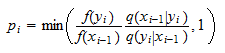3. draw a uniform random variable(on);

4. if, accept the proposal and set; otherwise, reject the proposal and set.

It can be proved that, provided some technical conditions are met, the sequencethus generated is the realization of a Markov Chain that converges to the stationary distribution. Furthermore, for any function,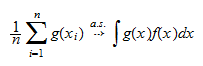whereis any function such that the expected valueexists and is finite anddenotes almost sure convergence astends to infinity. In other words, a Strong Law of Large Numbers (an ergodic theorem) holds for the sample mean.

The power of this algorithm lies in the fact that you need to know the functiononly up to a multiplicative constant. For example, in Bayesian inference it is very common to know the posterior distribution up to a multiplicative constant because the likelihood and the prior are known but the marginal distribution is not. Suppose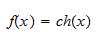and we knowbut not. Then, the acceptance probability in the M-H algorithm is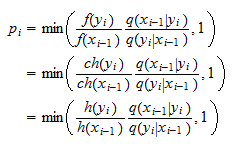As a consequence, the acceptance probability, which is the only quantity that depends on, can be computed without knowing the constant. This is the beauty of the Metropolis-Hastings algorithm: we can generate draws from a distribution even if we do not fully know the density of that distribution!

For more details see the lecture on the Metropolis-Hastings algorithm.

### Example 2 - Gibbs sampling

Another popular MCMC algorithm is the so-called Gibbs sampling algorithm.

Suppose you want to generate draws of a random vectorhaving joint density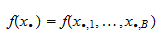whereareblocks of entries (or single entries) of.

Given a block, denote bythe vector comprising all blocks except.

Suppose you are able to generate draws from theconditional distributions, ...,. In MCMC jargon, these are called the full conditional distributions.

The Gibbs sampling algorithm starts from any vector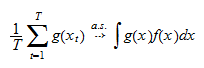belonging to the support of the target distribution and generates the subsequent valuesas follows:

1. for, generatefrom the conditional distribution with density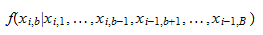In other words, at each iteration, the blocks are extracted one by one from their full conditional distributions, conditioning on the latest draws of all the other blocks.

Note that at iteration, when you extact the-th block, the latest draws of blockstoare those already extracted in iteration, while the latest draws of blockstoare those extracted in the previous iteration ().

It can be proved that Gibbs sampling is a special case of Metropolis-Hastings. Therefore, as in M-H, the sequencegenerated by the algorithm is the realization of a Markov Chain that converges to the stationary distribution. Furthermore, an ergodic theorem for the sample meansholds.

## Burn-in sample

A common practice is to discard the first draws of an MCMC sample. For example, if we have 110,000 draws, we discard the first 10,000 and keep the remaining 100,000.

The set of draws that are discarded is called the burn-in sample.

Why do we do this? If the starting valueis extracted from a distribution which is very different from the target distribution, then also the distributions of the subsequent draws,, ... will be very different from. However, the differences will become smaller and smaller because the chain converges to the target distribution. By discarding a burn-in sample, we eliminate draws whose distributions are far from the target distribution and we keep draws whose distributions are closer to the target. This reduces the bias of any Monte Carlo approximation performed with the MCMC sample.

The book

Most of the learning materials found on this website are now available in a traditional textbook format.

Glossary entries
Share